# How to Create Basic Ridgeline Plot in R

The ridgeline plot in R is used to visualize the distribution of a numerical value for several groups. Each distribution is presented as a line (often filled) and usually stacked over one another. This gives the appearance of mountain ridges, hence the name.

Ridgeline plots can be a helpful alternative to facetted histograms or density plots as they can save space and are visually engaging.

To create a ridgeline plot in R, use the “ggplot2 and ggridges packages.”

The ggplot2 library is essential for data visualization, while the ggridges library provides the functionality for the ridgeline plot.

Here is the step-by-step guide to create a basic Ridgeline plot in R.

## Step 1: Install and load the necessary libraries

``````install.packages("ggplot2")
install.packages("ggridges")
``````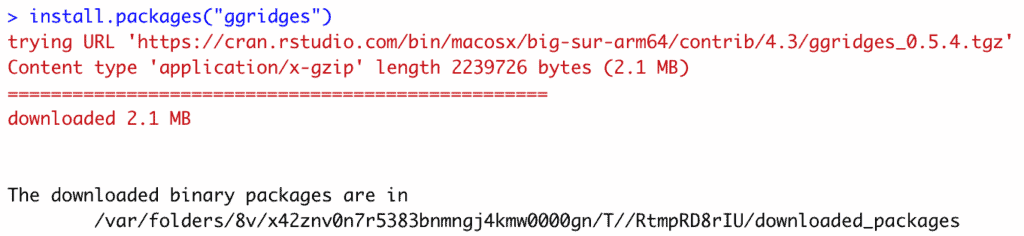I have already installed ggplot2 but not ggridges. That’s why I installed it, as you can see in the screenshot.

For this project, I will be using the “weather.csv” dataset.

Use the read.csv() function to import csv dataset and use it as a data frame in R.

``````# Load necessary libraries
library(ggplot2)
library(ggridges)

stringsAsFactors = FALSE)

# Display the first few rows of the dataset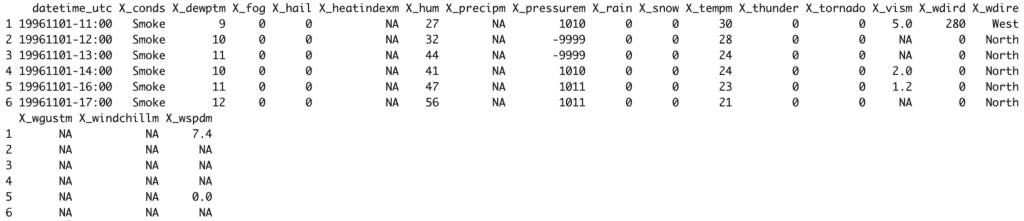We used the head() function to display the first six rows from the dataset to get an overview of the data.

## Step 3: Data Preprocessing

Before plotting, we need to process the data:

1. Extract the year from the datetime_utc column to create a new year column.
2. Handle missing values in the X_tempm column.
3. Filter out irrelevant years (e.g., if data for a year is sparse).

Let’s start by extracting the year:

``````# Extract the year from the datetime_utc column
weather_data\$year <- substr(weather_data\$datetime_utc, 1, 4)
``````

The next step is to handle missing values. A straightforward approach is to remove rows where the temperature is missing:

``````# Remove rows with NA values in the X_tempm column
weather_data <- weather_data[!is.na(weather_data\$X_tempm), ]
``````

## Step 4: Creating a ridgeline plot

``````# Load necessary libraries
library(ggplot2)
library(ggridges)

stringsAsFactors = FALSE)

# Extract the year from the datetime_utc column
weather_data\$year <- substr(weather_data\$datetime_utc, 1, 4)

# Remove rows with NA values in the X_tempm column
weather_data <- weather_data[!is.na(weather_data\$X_tempm), ]

# Create the ridgeline plot
ggplot(weather_data, aes(x = X_tempm, y = as.factor(year), fill = ..density..)) +
geom_density_ridges_gradient(scale = 3, rel_min_height = 0.01) +
labs(title = "Temperature Distribution Over Years", x = "Temperature (°C)", y = "Year") +
theme_ridges(font_size = 13, grid = TRUE)``````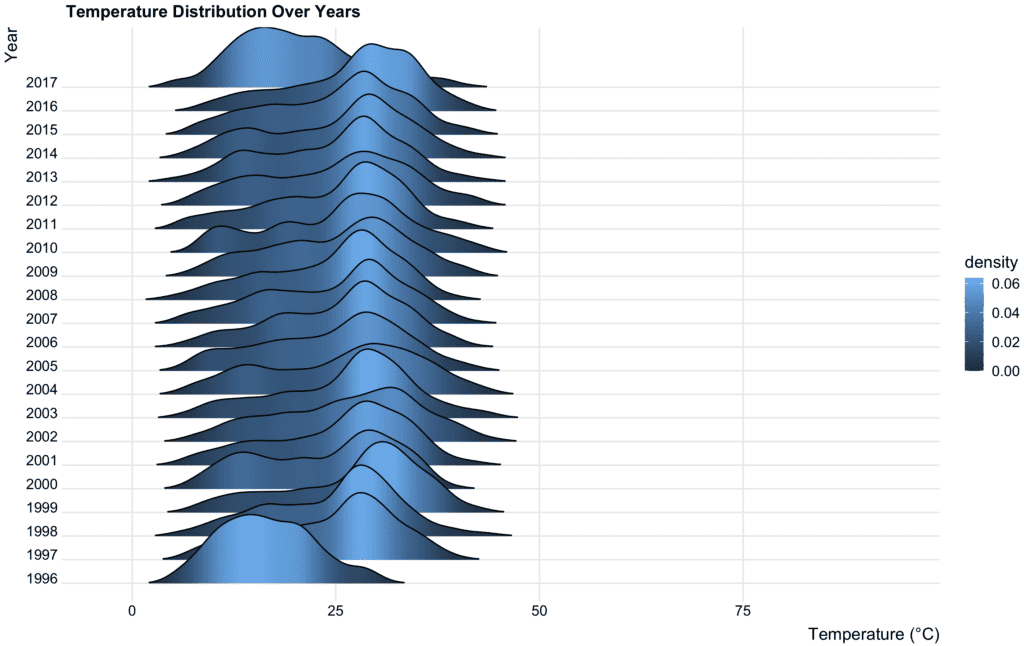## geom_density_ridges() function

The geom_density_ridges() function from the ggridges package in R is used to visualize the distribution of a numerical variable across different categories. It’s helpful when you want to compare these distributions side-by-side.

``````ggplot(weather_data, aes(x = X_tempm, y = as.factor(year))) +
geom_density_ridges() +
labs(title = "Temperature Distribution Over Years",
x = "Temperature (°C)",
y = "Year")``````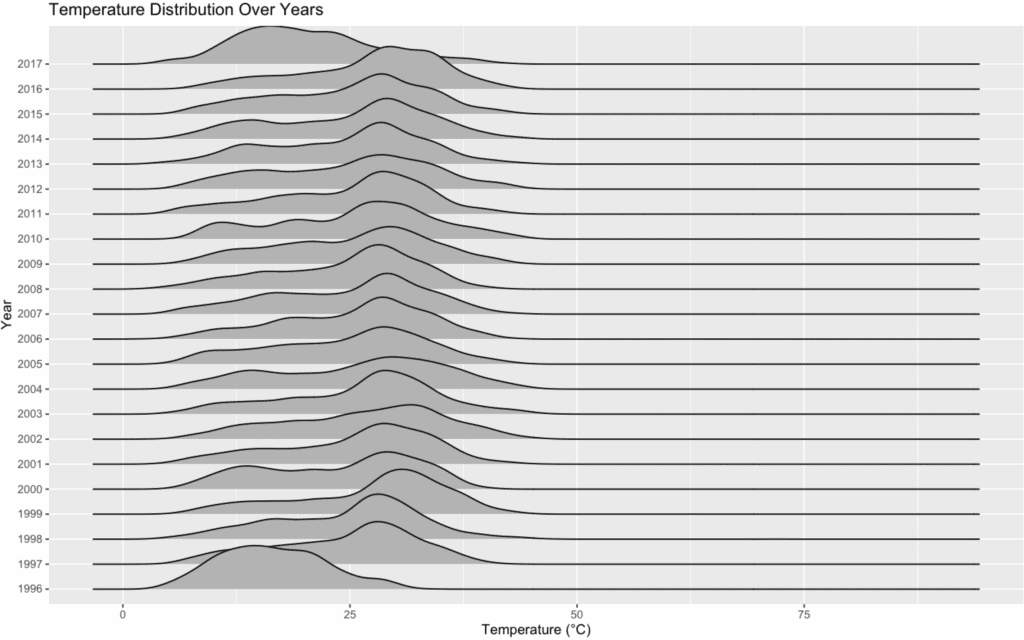## Cut the trailing tails

The rel_min_height argument of the geom_density_ridges() function is used to cut the trailing tails.

For instance:

1. If you set rel_min_height = 0.05, it will cut off any parts of the density ridge below 5% of the maximum height of that ridge.
2. If you set rel_min_height = 0, no parts will be cut off, and you will see the full-density ridge.

Fine-tuning the value of rel_min_height is somewhat subjective and depends on the dataset and the specific visual representation you aim for.

``````ggplot(weather_data, aes(x = X_tempm, y = as.factor(year), fill = ..density..)) +
geom_density_ridges_gradient(rel_min_height = 0.05, scale = 3) +
labs(title = "Temperature Distribution Over Years", x = "Temperature (°C)", y = "Year") +
theme_ridges(font_size = 13, grid = TRUE)
``````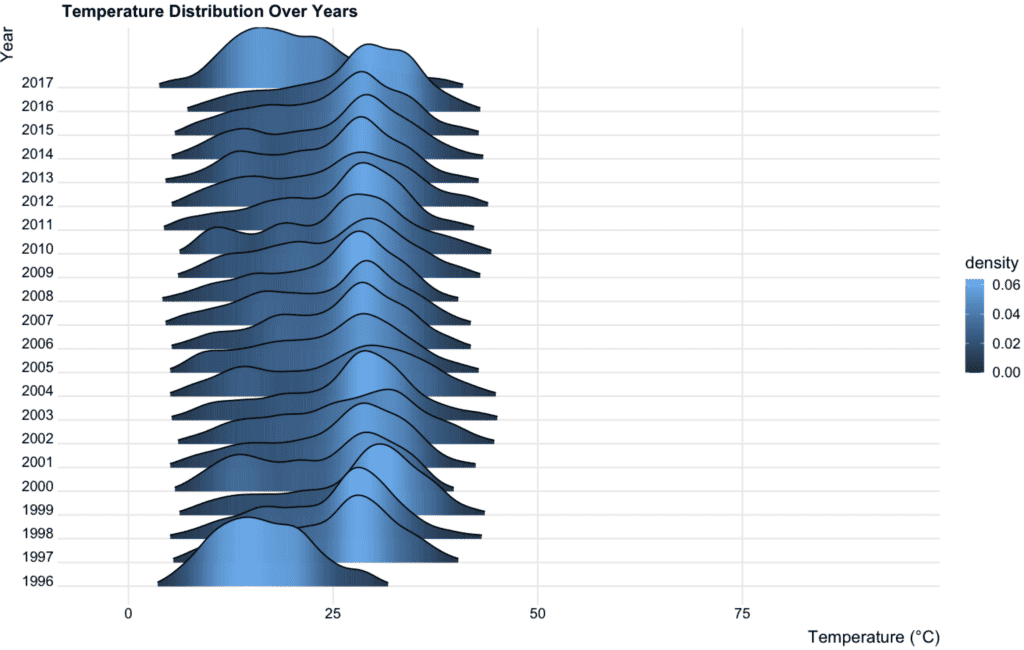## Scaling

The scale argument in the geom_density_ridges() function defines the scaling of the density ridges relative to the spacing between them.

It controls how much the ridges overlap or how separated they appear.

1. scale = 1 (the default) means that the maximum height of each ridge equals the minimum spacing between the groups (or ridges).
2. scale > 1 will increase the height of the ridges, causing them to overlap more.
3. scale < 1 will decrease the height, separating the ridges more.

Adjusting the scale parameter can emphasize the differences between groups or allow for a more compact visual representation.

``````ggplot(weather_data, aes(x = X_tempm, y = as.factor(year), fill = ..density..)) +
geom_density_ridges_gradient(scale = 2, rel_min_height = 0.05) +
labs(title = "Temperature Distribution Over Years", x = "Temperature (°C)", y = "Year") +
theme_ridges(font_size = 13, grid = TRUE)``````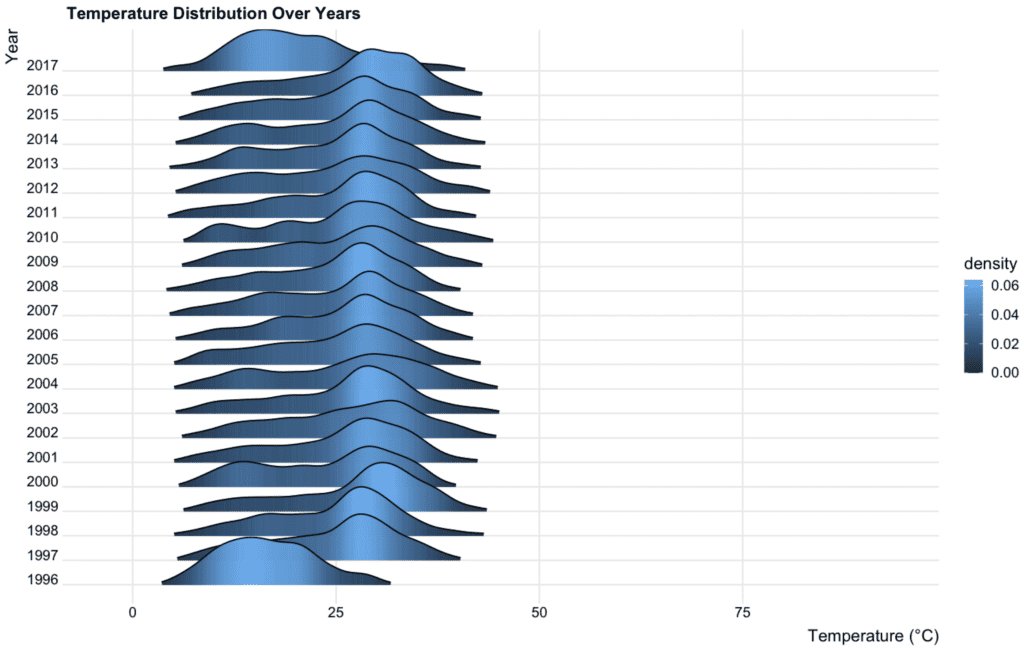In a ridgeline plot, each “ridge” represents the density distribution of a numerical variable for a specific category.

Shape variation is evident when these density distributions (or ridges) differ in their appearance across categories.

When analyzing shape variation, it’s also essential to consider external factors that might influence the data.

For instance, in our temperature dataset, factors like changes in measurement techniques, equipment, or location can influence temperature readings and should be accounted for when concluding.

You can check out the GitHub code.

## What to do next?

If you are interested in other aspects of the weather data, you can also create additional visualizations, such as:

1. A time series plot for temperature or humidity over the years.
2. A bar plot showing the frequency of different weather conditions (X_conds).

That’s all!